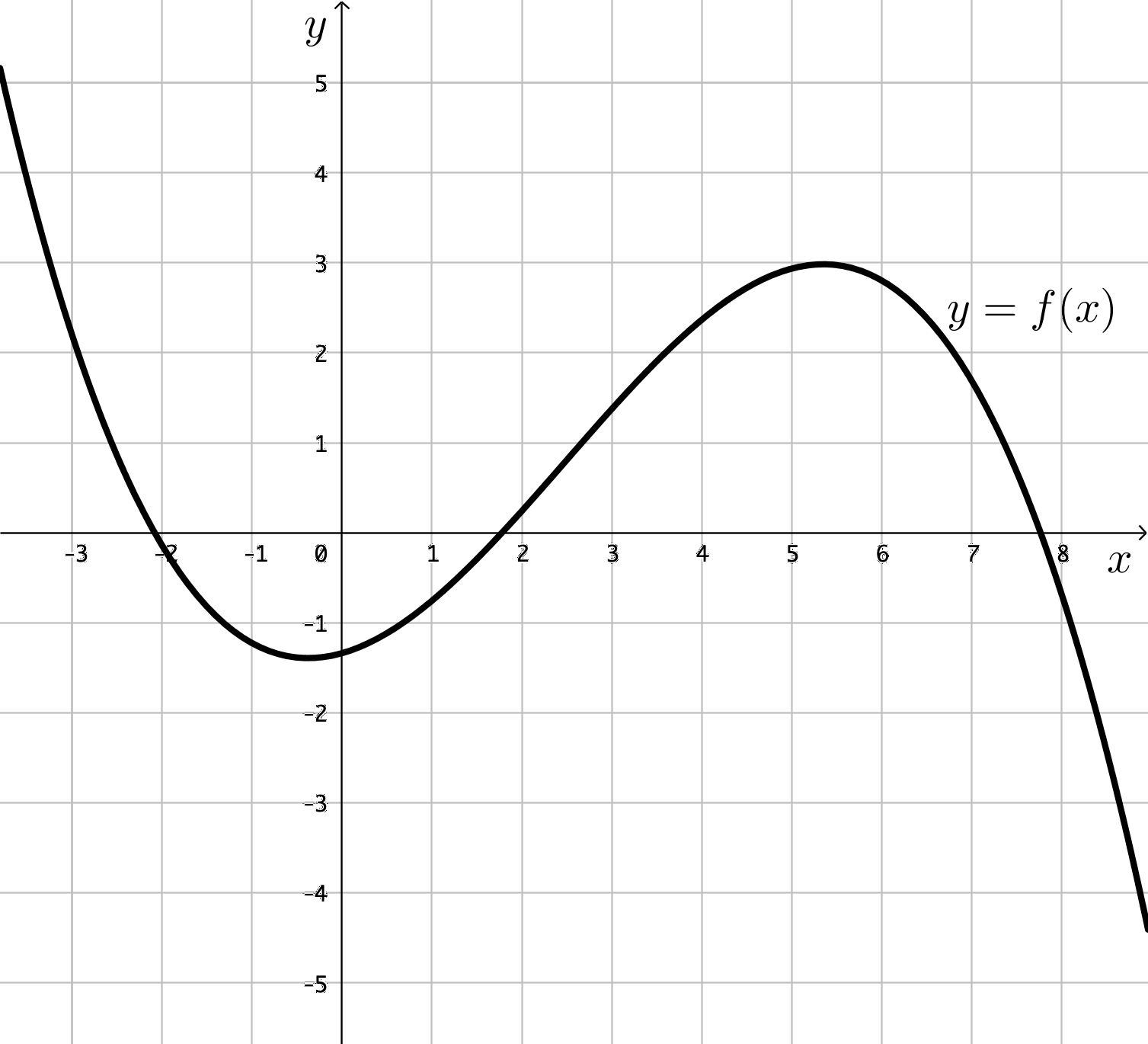$\newenvironment {prompt}{}{} \newcommand {\ungraded }{} \newcommand {\HyperFirstAtBeginDocument }{\AtBeginDocument }$

For Questions 1 and 2 use the following graphs:

 Graph IGraph IIGraph IIIGraph IVGraph VFor which of the graphs from I) – V) above, if any, is there a value for $c$ in $(-2,7)$ where $f'(c) = \dfrac {f(7)-f(-2)}{7-(-2)}$?
Graph I) only Graph II) only Graph III) only Graph IV) only Graph V) only Graph III) and Graph IV) only Graph IV) and Graph V) only Graph I), Graph IV) and Graph V) only Graph III), Graph IV) and Graph V) only Graph I), Graph II), Graph IV), and Graph V) only Graph I), Graph III), Graph IV), and Graph V) only All five functions have a value for $c$ in $(-2,7)$ where $f'(c) = \dfrac {f(7)-f(-2)}{7-(-2)}$ None of the graphs have a value for $c$ in $(-2,7)$ where $f'(c) = \dfrac {f(7)-f(-2)}{7-(-2)}$
For which of the graphs from I) – V) above, if any, is continuous over $[-2,7]$, differentiable over $(-2,7)$, AND there is a value for $c$ in $(-2,7)$ where $f'(c) = \dfrac {f(7)-f(-2)}{7-(-2)}$?
Graph I) only Graph II) only Graph III) only Graph IV) only Graph V) only Graph III) and Graph IV) only Graph IV) and Graph V) only Graph I), Graph IV) and Graph V) only Graph III), Graph IV) and Graph V) only Graph I), Graph II), Graph IV), and Graph V) only Graph I), Graph III), Graph IV), and Graph V) only All five functions satisfy these three conditions None of the graphs have functions that satisfy these three conditions
For the graph of $y = f(x)$ below, identify a value for $c$, if any, from the interval $(-3,7)$ where $f'(c)=\dfrac {f(7)-f(-3)}{7-(-3)}$.
$c=0$ $c=0.5$ $c=2$ $c=4$ $c=4.4$ $c=5.2$ No values for $c$ exist where $f'(c)=\dfrac {f(7)-f(-3)}{7-(-3)}$
Two highway patrol cars are stationed 4.2 miles apart on an interstate highway that has a speed limit of 65 miles per hour. A gray SUV passes in front of the first patrol car and is clocked at 63 miles per hour. 0.062 hours later (almost 4 minutes), the gray SUV passes in front of the second patrol car and is clocked at 64 miles per hour. Did the gray SUV exceed the speed limit at some time during the 0.062 hours?